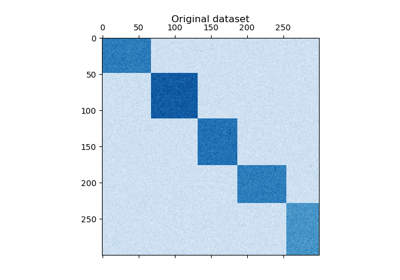# sklearn.cluster.SpectralCoclustering¶

class sklearn.cluster.SpectralCoclustering(n_clusters=3, *, svd_method='randomized', n_svd_vecs=None, mini_batch=False, init='k-means++', n_init=10, n_jobs='deprecated', random_state=None)[source]

Spectral Co-Clustering algorithm (Dhillon, 2001).

Clusters rows and columns of an array X to solve the relaxed normalized cut of the bipartite graph created from X as follows: the edge between row vertex i and column vertex j has weight X[i, j].

The resulting bicluster structure is block-diagonal, since each row and each column belongs to exactly one bicluster.

Supports sparse matrices, as long as they are nonnegative.

Read more in the User Guide.

Parameters
n_clustersint, default=3

The number of biclusters to find.

svd_method{‘randomized’, ‘arpack’}, default=’randomized’

Selects the algorithm for finding singular vectors. May be ‘randomized’ or ‘arpack’. If ‘randomized’, use sklearn.utils.extmath.randomized_svd, which may be faster for large matrices. If ‘arpack’, use scipy.sparse.linalg.svds, which is more accurate, but possibly slower in some cases.

n_svd_vecsint, default=None

Number of vectors to use in calculating the SVD. Corresponds to ncv when svd_method=arpack and n_oversamples when svd_method is ‘randomized.

mini_batchbool, default=False

Whether to use mini-batch k-means, which is faster but may get different results.

init{‘k-means++’, ‘random’, or ndarray of shape (n_clusters, n_features), default=’k-means++’

Method for initialization of k-means algorithm; defaults to ‘k-means++’.

n_initint, default=10

Number of random initializations that are tried with the k-means algorithm.

If mini-batch k-means is used, the best initialization is chosen and the algorithm runs once. Otherwise, the algorithm is run for each initialization and the best solution chosen.

n_jobsint, default=None

The number of jobs to use for the computation. This works by breaking down the pairwise matrix into n_jobs even slices and computing them in parallel.

None means 1 unless in a joblib.parallel_backend context. -1 means using all processors. See Glossary for more details.

Deprecated since version 0.23: n_jobs was deprecated in version 0.23 and will be removed in 0.25.

random_stateint, RandomState instance, default=None

Used for randomizing the singular value decomposition and the k-means initialization. Use an int to make the randomness deterministic. See Glossary.

Attributes
rows_array-like of shape (n_row_clusters, n_rows)

Results of the clustering. rows[i, r] is True if cluster i contains row r. Available only after calling fit.

columns_array-like of shape (n_column_clusters, n_columns)

Results of the clustering, like rows.

row_labels_array-like of shape (n_rows,)

The bicluster label of each row.

column_labels_array-like of shape (n_cols,)

The bicluster label of each column.

References

Examples

>>> from sklearn.cluster import SpectralCoclustering
>>> import numpy as np
>>> X = np.array([[1, 1], [2, 1], [1, 0],
...               [4, 7], [3, 5], [3, 6]])
>>> clustering = SpectralCoclustering(n_clusters=2, random_state=0).fit(X)
>>> clustering.row_labels_ #doctest: +SKIP
array([0, 1, 1, 0, 0, 0], dtype=int32)
>>> clustering.column_labels_ #doctest: +SKIP
array([0, 0], dtype=int32)
>>> clustering
SpectralCoclustering(n_clusters=2, random_state=0)


Methods

 fit(X[, y]) Creates a biclustering for X. Row and column indices of the i’th bicluster. get_params([deep]) Get parameters for this estimator. Shape of the i’th bicluster. get_submatrix(i, data) Return the submatrix corresponding to bicluster i. set_params(**params) Set the parameters of this estimator.
__init__(n_clusters=3, *, svd_method='randomized', n_svd_vecs=None, mini_batch=False, init='k-means++', n_init=10, n_jobs='deprecated', random_state=None)[source]

Initialize self. See help(type(self)) for accurate signature.

property biclusters_

Convenient way to get row and column indicators together.

Returns the rows_ and columns_ members.

fit(X, y=None)[source]

Creates a biclustering for X.

Parameters
Xarray-like, shape (n_samples, n_features)
yIgnored
get_indices(i)[source]

Row and column indices of the i’th bicluster.

Only works if rows_ and columns_ attributes exist.

Parameters
iint

The index of the cluster.

Returns
row_indndarray, dtype=np.intp

Indices of rows in the dataset that belong to the bicluster.

col_indndarray, dtype=np.intp

Indices of columns in the dataset that belong to the bicluster.

get_params(deep=True)[source]

Get parameters for this estimator.

Parameters
deepbool, default=True

If True, will return the parameters for this estimator and contained subobjects that are estimators.

Returns
paramsmapping of string to any

Parameter names mapped to their values.

get_shape(i)[source]

Shape of the i’th bicluster.

Parameters
iint

The index of the cluster.

Returns
shapetuple (int, int)

Number of rows and columns (resp.) in the bicluster.

get_submatrix(i, data)[source]

Return the submatrix corresponding to bicluster i.

Parameters
iint

The index of the cluster.

dataarray-like

The data.

Returns
submatrixndarray

The submatrix corresponding to bicluster i.

Notes

Works with sparse matrices. Only works if rows_ and columns_ attributes exist.

set_params(**params)[source]

Set the parameters of this estimator.

The method works on simple estimators as well as on nested objects (such as pipelines). The latter have parameters of the form <component>__<parameter> so that it’s possible to update each component of a nested object.

Parameters
**paramsdict

Estimator parameters.

Returns
selfobject

Estimator instance.

## Examples using sklearn.cluster.SpectralCoclustering`¶